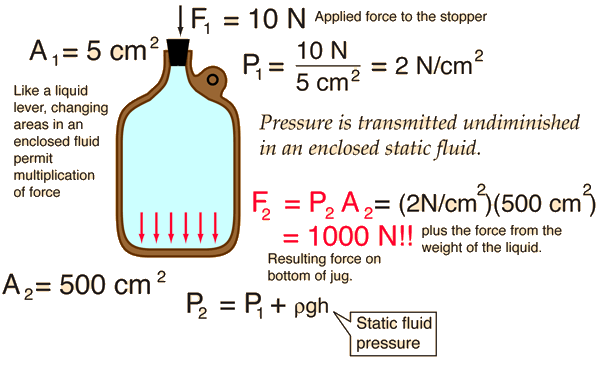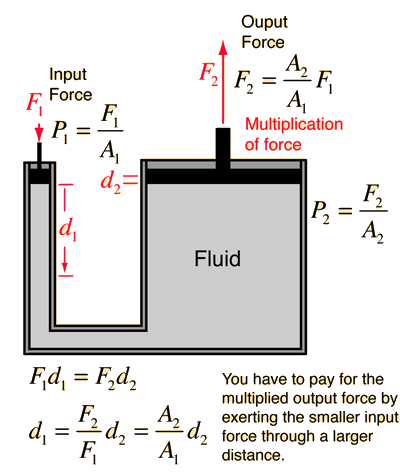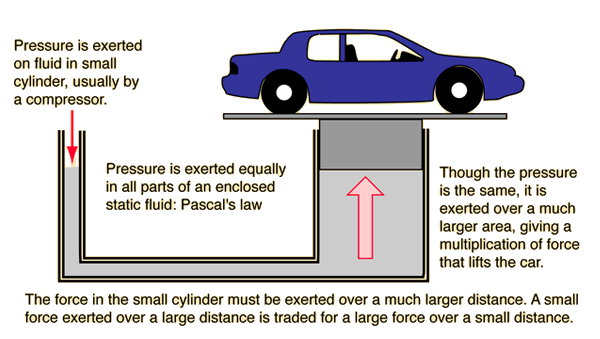Pascal's Principle

Pressure is transmitted undiminished in an enclosed static fluid.Any externally applied pressure is transmitted to all parts of the enclosed fluid, making possible a large multiplication of force (hydraulic press principle). The pressure at the bottom of the jug is equal to the externally applied pressure on the top of the fluid plus the static fluid pressure from the weight of the liquid.

How to drop the bottom out of a bottle

Index

Pascal's principle concepts

 HyperPhysics***** Mechanics ***** Fluids R Nave
Go Back

Hydraulic Press

A multiplication of force can be achieved by the application of fluid pressure according to Pascal's principle, which for the two pistons implies

P1 = P2

This allows the lifting of a heavy load with a small force, as in an auto hydraulic lift, but of course there can be no multiplication of work, so in an ideal case with no frictional loss:

Winput = Woutput

CalculationIndex

Pascal's principle concepts

 HyperPhysics***** Mechanics ***** Fluids R Nave
Go Back

Hydraulic Press Calculation

 Example of hydraulic press action. For piston radii: r1 = cm r1 = in and r2 = cm r2 = in the cylinder areas are A1 = m2 A2 = m2 and the force multiplication is A2/A1 = For an input force F1 = N F1 = lb, Pascal's principle gaurantees that P1 = P2 = kPa P1 = P2 = lb/in2.Applying this pressure to the output cylinder yields the lifting force:

F2 = N = lb,

A price must be paid for an increased lifting force, since conservation of energy must be satisfied. For an ideal hydraulic press, the work must satisfy Winput = Wouput, so that to lift the load a distance of

d2 = m = ft,
the input piston must be moved a distance
d1 = m = ft,
Index

Pascal's principle concepts

 HyperPhysics***** Mechanics ***** Fluids R Nave
Go Back

Automobile Hydraulic Lift

A hydraulic lift for automobiles is an example of a force multiplied by hydraulic press, based on Pascal's principle. The fluid in the small cylinder must be moved much further than the distance the car is lifted.For example, if the lift cylinder were 25 cm in diameter and the small cylinder were 1.25 cm in diameter, then the ratio of the areas is 400, so the hydraulic press arrangement gives a multiplication of 400 times the force. To lift a 6000 newton car, you would have to exert only 6000 N/400 = 15 N on the fluid in the small cylinder to lift the car. However, to lift the car 10 cm, you would have to move the oil 400 x 10cm = 40 meters. This is practical by pumping oil into this small cylinder with a small compressor.

Calculation

Index

Pascal's principle concepts

 HyperPhysics***** Mechanics ***** Fluids R Nave
Go Back